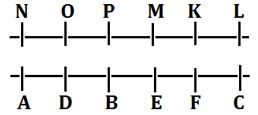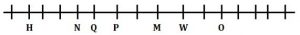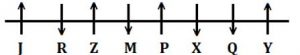Latest Banking jobs   »

# Reasoning Ability Quiz For FCI Phase I 2022- 19th September

Directions (1-5): Study the following information to answer the given questions:
Twelve people are sitting in two parallel rows containing six people each in such a way that there is an equal distance between adjacent persons. In row-1, K, L, M, N, O and P are sitting and all of them are facing South. In row-2, A, B, C, D, E and F are sitting and all of them are facing North. Therefore, in the given seating arrangement, each member of a row faces another member of the other row.
C sits third to the right of B. B faces P and P does not sit at any of the extreme ends of the lines. N sits third to the right of M. F faces K. The one facing K sits third to the right of D. O and D does not sit at the extreme ends of the lines. A is not an immediate neighbour of B and P is not an immediate neighbour of N.

Q1. Who among the following sits opposite to M?
(a) D
(b) C
(c) E
(d) B
(e) None of these

Q2. How many persons sit between A and F?
(a) One
(b) Two
(c) More than Three
(d) Three
(e) None

Q3. Who among the following sits third to the left of P?
(a) O
(b) L
(c) K
(d) N
(e) None of these

Q4. What is the position of E with respect to D?
(a) Second to the right
(b) Immediate left
(c) Third to the right
(d) Second to the left
(e) None of these

Q5. Which among the following persons sit opposite to the one who sits second to the right of M?
(a) E
(b) D
(c) F
(d) C
(e) None of these

Directions (6-10): Study the information carefully and answer the questions given below.
An uncertain number of persons sit in a linear row all are facing north direction. Four persons sit between P and H.Q sits fourth to the right of H. Three persons sit between Q and W. N sits fifth to the left of W. O sits fifth from one of the extreme ends of row and sit second to the right of W. M sits third to the left of O. H sits second from one of the extreme ends of row.

Q6. How many persons sit in the row?
(a) 16
(b) 18
(c) 19
(d) 20
(e) None of these

Q7. Who among the following person sit immediate right of M?
(a) O
(b) N
(c) Q
(d) P
(e) None of these

Q8. How many persons sit between P and O?
(a) Two
(b) One
(c) Three
(d) Four
(e) None

Q9. If L sits immediate right of O, then what is the position of L with respect to Q?
(a) Sixth to the left
(b) Fourth to the left
(c) Fifth to the right
(d) Seventh to the right
(e) None of these

Q10. Which of the following statement is true regarding N?
(a) N sits second to the right of P
(b) One person sits between H and N
(c) N sits immediate left of Q
(d) More than three persons sit between Na and M
(e) None is true

Directions (11-15): Study the information carefully and answer the questions given below.
Eight persons are sitting in a row some of them facing north and some of them facing south. M sits third to the right of J, who sits one of the extreme ends of the row. Six persons sit to the right of Q. Z sits fourth to the right of Q. Immediate neighbor of Z face same direction to each other’s. Both X and P sits immediate right to each other’s. One person sits between P and Z. P sits third to the left of R. R is not immediate neighbor of Q and M. Not more than four persons face south direction. P sits third to the left of Y. M does not face North.

Q11.Who among the following person sits immediate left of Z?
(a) R
(b) M
(c) J
(d) X
(e) None of these

Q12. How many persons sits between Z and Q?
(a) Two
(b) One
(c) Three
(d) Four
(e) None

Q13. How many persons face north?
(a) Six
(b) Five
(c) Three
(d) Four
(e) Two

Q14. Four of the following five are alike in certain way based from a group, find the one that does not belong to that group?
(a) X
(b) Q
(c) M
(d) Y
(e) R

Q15. Who among the following sits immediate right of Y?
(a) Q
(b) X
(c) Z
(d) M
(e) No one

Solutions

Solutions (1-5):
Sol.S1. Ans. (c)
S2. Ans. (d)
S3. Ans. (b)
S4. Ans. (a)
S5. Ans. (b)

Solutions (6-10):
Sol.S6. Ans. (a)
S7. Ans. (e)
S8. Ans. (d)
S9. Ans. (d)
S10. Ans. (c)

Solutions (11-15):
Sol.S11. Ans. (a)
S12. Ans. (c)
S13. Ans. (d)
S14. Ans. (d)
S15. Ans. (e)#### Congratulations!Download Hindu Review of October 2021: Free PDF